# How to Calculate and Solve for Hydrostatic Pressure of a Dam | Water Budget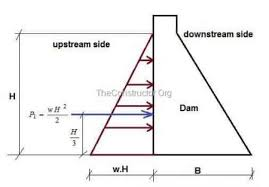The image above represents hydrostatic pressure of a dam.

To compute for hydrostatic pressure of a dam, two essential parameters are needed and these parameters are Specific Weight of Water (γ) and Depth of water (h).

The formula for calculating hydrostatic pressure of a dam:

Hn = γh² / 2

Where:

Hn = Hydrostatic Pressure of a Dam
γ = Specific Weight of Water
h = Depth of Water

Let’s solve an example;
Find the hydrostatic pressure of a dam when the specific weight of water is 10 and the depth of water is 5.

This implies that;

γ = Specific Weight of Water = 10
h = Depth of Water = 5

Hn = γh² / 2
Hn = (10)(5)² / 2
Hn = 10(25) / 2
Hn = 250 / 2
Hn = 125

Therefore, the hydrostatic pressure of the dam is 125.

Calculating the Specific Weight of Water when the Hydrostatic Pressure of the Dam and the Depth of Water is Given.

γ = Hn x 2 / h2

Where;

γ = Specific Weight of Water
Hn = Hydrostatic Pressure of a Dam
h = Depth of Water

Let’s solve an example;
Find the specific weight of water when the hydrostatic pressure of a dam is 30 and the depth of water is 6.

This implies that;

Hn = Hydrostatic Pressure of a Dam = 30
h = Depth of Water = 6

γ = Hn x 2 / h2
γ = 30 x 2 / 62
γ = 60 / 36
γ = 1.667

Therefore, the specific weight of water is 1.667.

Calculating the Depth of Water when the Hydrostatic Pressure of the Dam and the Specific Weight of Water is Given.

h = √Hn x 2 / γ

Where;

h = Depth of Water
Hn = Hydrostatic Pressure of a Dam
γ = Specific Weight of Water

Let’s solve an example;
Find the depth of water when the hydrostatic pressure of a dam is 12 and the specific weight of water is 4.

This implies that;

Hn = Hydrostatic Pressure of a Dam = 12
γ = Specific Weight of Water = 4

h = √Hn x 2 / γ
h = √12 x 2 / 4
h = √24 / 4
h = √6
h = 2.449

Therefore, the depth of water is 2.449.

Nickzom Calculator – The Calculator Encyclopedia is capable of calculating the depth of water.

To get the answer and workings of the depth of water using the Nickzom Calculator – The Calculator Encyclopedia. First, you need to obtain the app.

You can get this app via any of these means:

To get access to the professional version via web, you need to register and subscribe for NGN 1,500 per annum to have utter access to all functionalities.
You can also try the demo version via https://www.nickzom.org/calculator

Apple (Paid) – https://itunes.apple.com/us/app/nickzom-calculator/id1331162702?mt=8
Once, you have obtained the calculator encyclopedia app, proceed to the Calculator Map, then click on Agricultural under Engineering.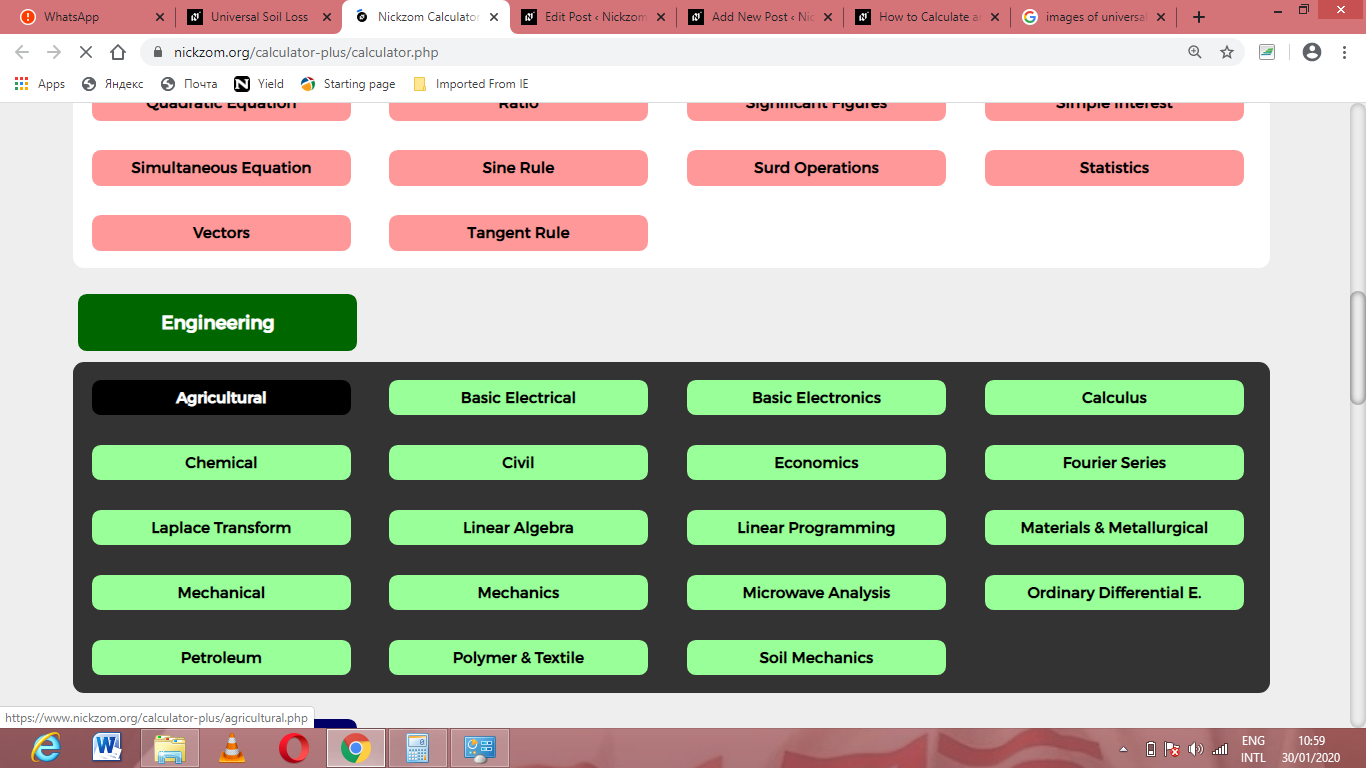Now, Click on Water Budget under Agricultural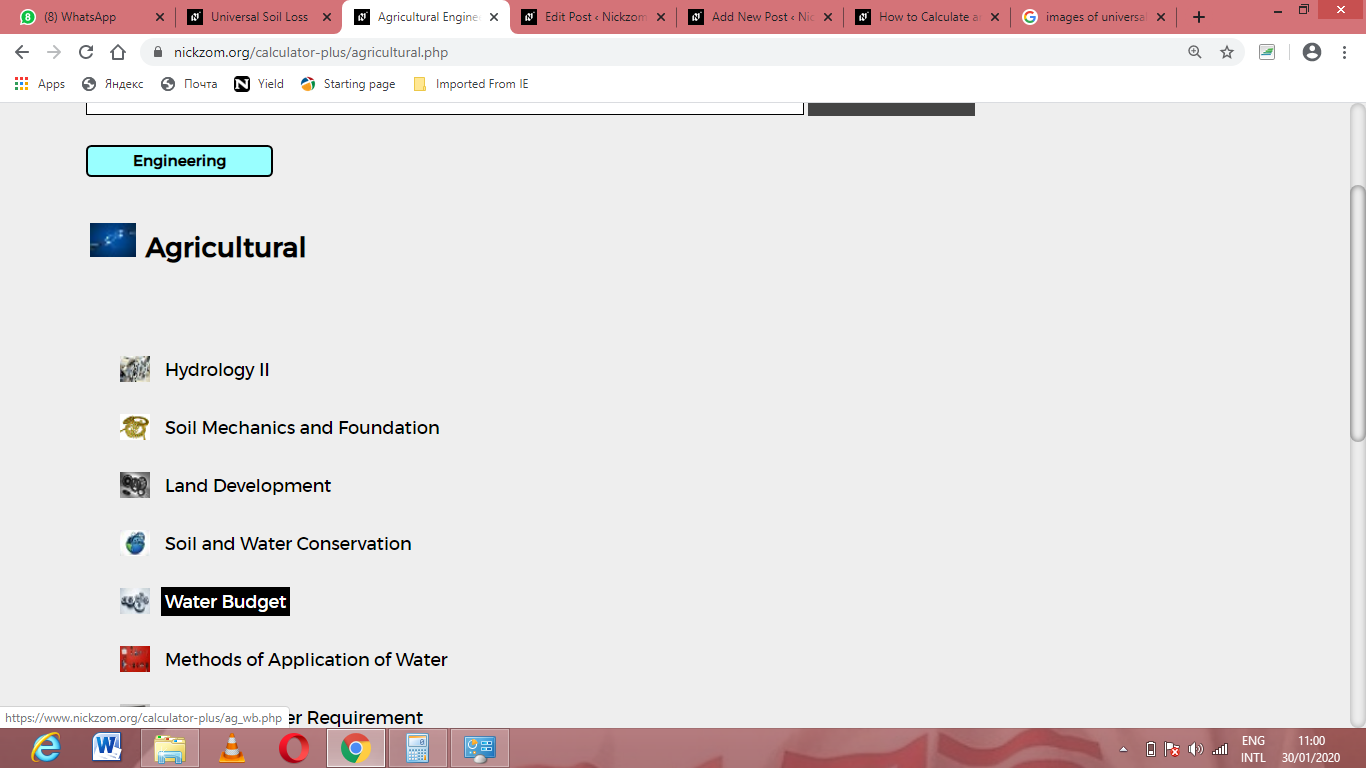Now, Click on Hydrostatic Pressure of a Dam under Water Budget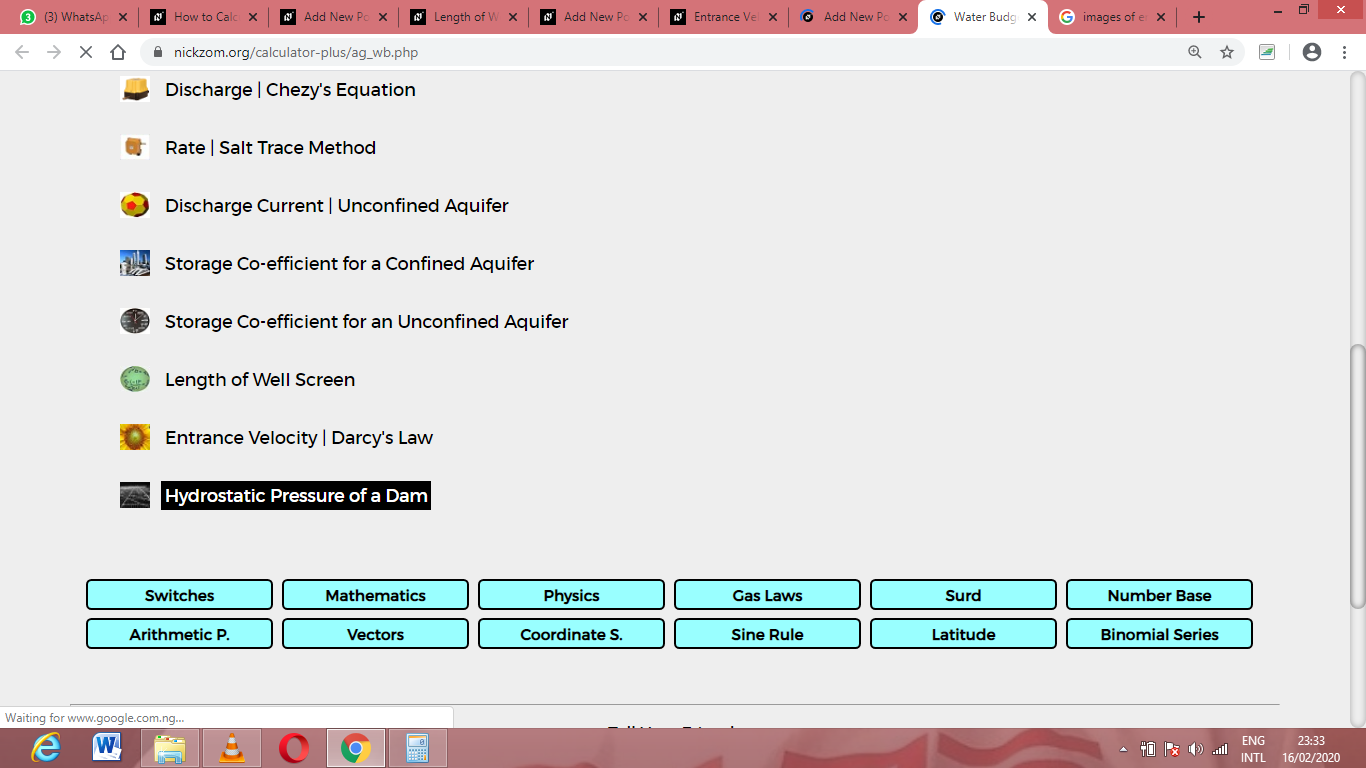The screenshot below displays the page or activity to enter your values, to get the answer for the hydrostatic pressure of a dam according to the respective parameters which are the Specific Weight of Water (γ) and Depth of water (h).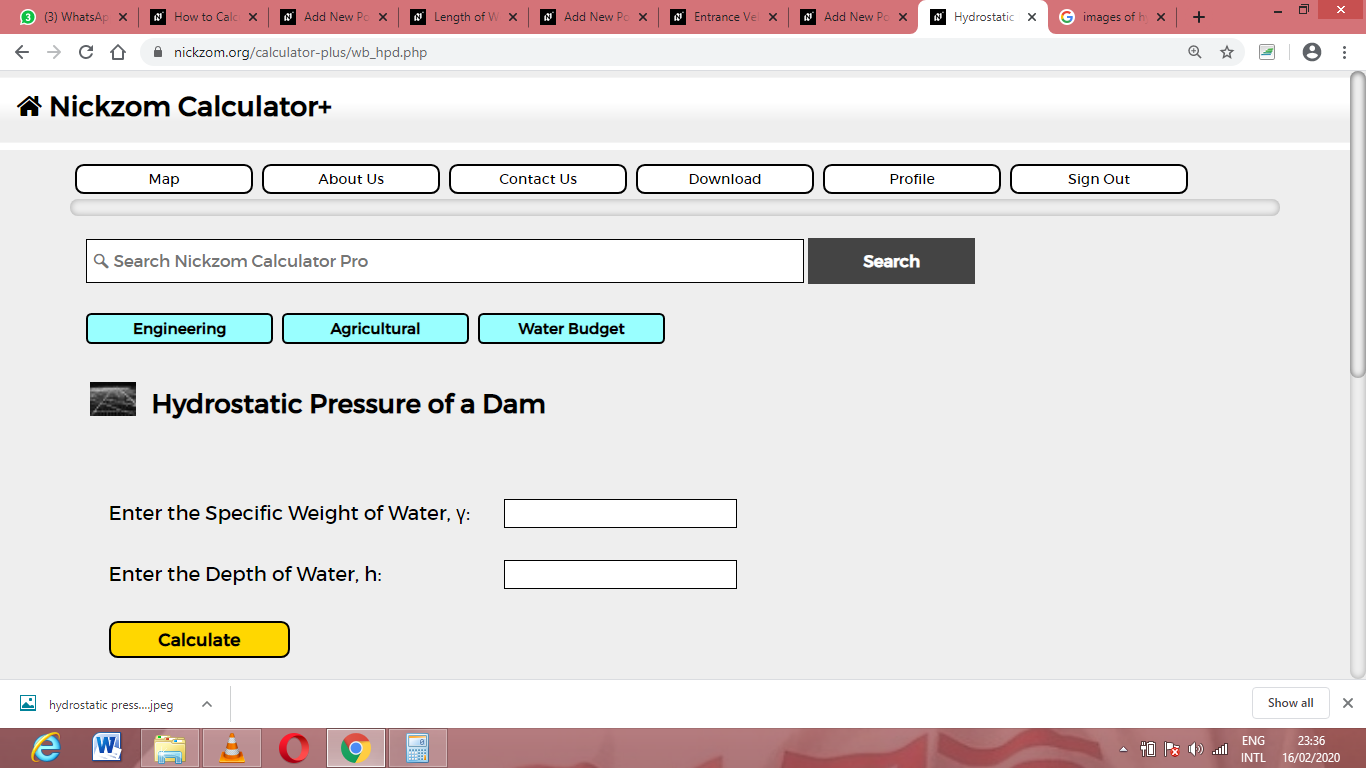Now, enter the values appropriately and accordingly for the parameters as required by the Specific Weight of Water (γ) is 10 and Depth of water (h) is 5.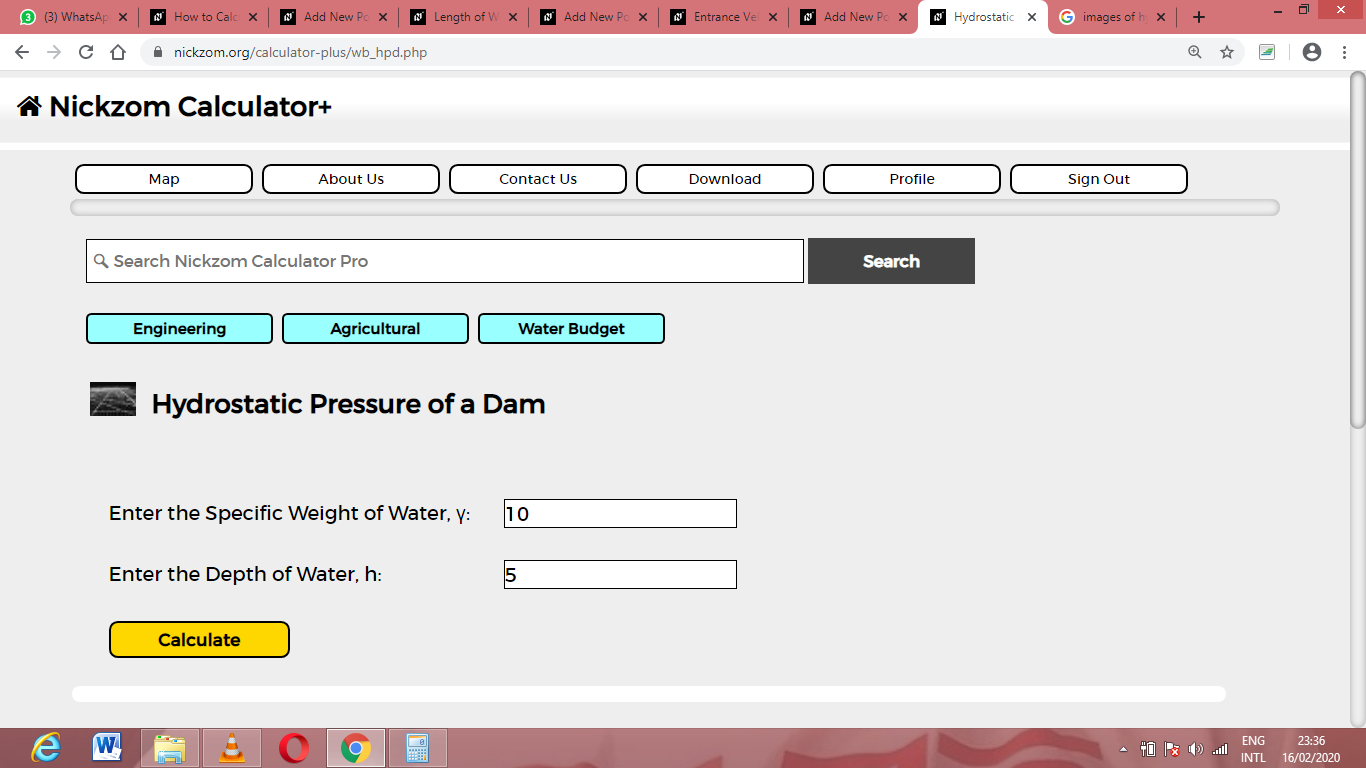Finally, Click on Calculate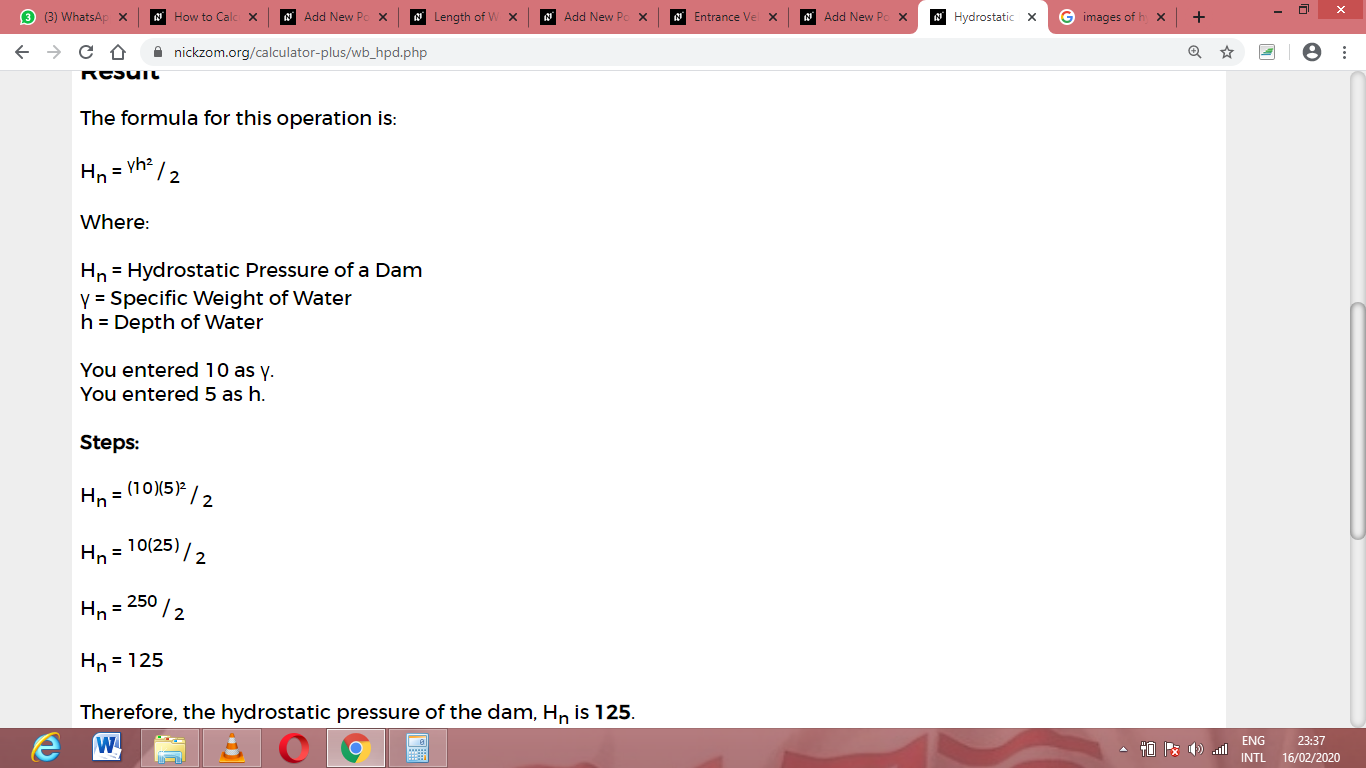As you can see from the screenshot above, Nickzom Calculator– The Calculator Encyclopedia solves for the hydrostatic pressure of a dam and presents the formula, workings and steps too.